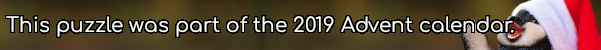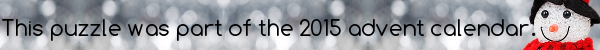mscroggs.co.uk
mscroggs.co.uksubscribe

# Puzzles

## 19 DecemberThe diagram below shows three squares and five circles. The four smaller circles are all the same size, and the red square's vertices are the centres of these circles.
The area of the blue square is 14 units. What is the area of the red square?

## 5 December28 points are spaced equally around the circumference of a circle. There are 3276 ways to pick three of these points. The three picked points can be connected to form a triangle. Today's number is the number of these triangles that are isosceles.

## 2 DecemberToday's number is the area of the largest dodecagon that it's possible to fit inside a circle with area $$\displaystyle\frac{172\pi}3$$.

## Two semicircles

The diagram shows two semicircles.
$$CD$$ is a chord of the larger circle and is parallel to $$AB$$. The length of $$CD$$ is 8m. What is the area of the shaded region (in terms of $$\pi$$)?

## 1 DecemberWhat is area of the largest area rectangle which will fit in a circle of radius 10?

## Squared circle

Each side of a square has a circle drawn on it as diameter. The square is also inscribed in a fifth circle as shown.
Find the ratio of the total area of the shaded crescents to the area of the square.

## Dartboard

Concentric circles with radii 1, $$\frac{1}{2}$$, $$\frac{1}{3}$$, $$\frac{1}{4}$$, ... are drawn. Alternate donut-shaped regions are shaded.
What is the total shaded area?

## Circles

Which is largest, the red or the blue area?

## Archive

Show me a random puzzle
▼ show ▼